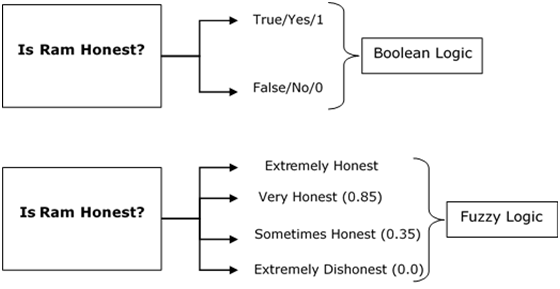# Fuzzy Logic - Introduction

The word fuzzy refers to things which are not clear or are vague. Any event, process, or function that is changing continuously cannot always be defined as either true or false, which means that we need to define such activities in a Fuzzy manner.

## What is Fuzzy Logic?

Fuzzy Logic resembles the human decision-making methodology. It deals with vague and imprecise information. This is gross oversimplification of the real-world problems and based on degrees of truth rather than usual true/false or 1/0 like Boolean logic.

Take a look at the following diagram. It shows that in fuzzy systems, the values are indicated by a number in the range from 0 to 1. Here 1.0 represents absolute truth and 0.0 represents absolute falseness. The number which indicates the value in fuzzy systems is called the truth value.In other words, we can say that fuzzy logic is not logic that is fuzzy, but logic that is used to describe fuzziness. There can be numerous other examples like this with the help of which we can understand the concept of fuzzy logic.

Fuzzy Logic was introduced in 1965 by Lofti A. Zadeh in his research paper “Fuzzy Sets”. He is considered as the father of Fuzzy Logic.